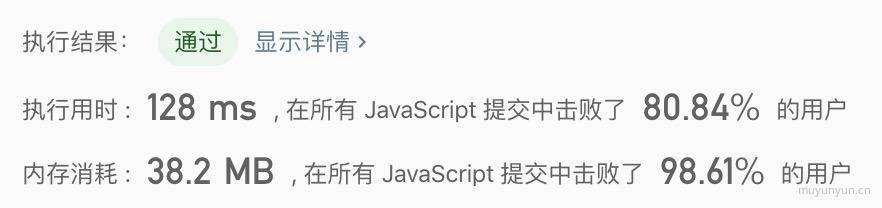Time FlyingYou are given two non-empty linked lists representing two non-negative integers. The most significant digit comes first and each of their nodes contain a single digit. Add the two numbers and return it as a linked list.

You may assume the two numbers do not contain any leading zero, except the number 0 itself.

What if you cannot modify the input lists? In other words, reversing the lists is not allowed.

Example:

Input: (7 -> 2 -> 4 -> 3) + (5 -> 6 -> 4)Output: 7 -> 8 -> 0 -> 7

### Analyze

• 第一步: 首先补齐位数, 让其一一对应;
7  ->  2  ->  4  ->  30  ->  5  ->  6  ->  4
• 第二步: 递归计算两个链表同位之和, 同时使用 digitCarry 表示进位的情况;
/** * Definition for singly-linked list. * function ListNode(val) { *     this.val = val; *     this.next = null; * } *//** * @param {ListNode} l1 * @param {ListNode} l2 * @return {ListNode} */var addTwoNumbers = function(l1, l2) {  let countl1 = 0, countl2 = 0  let l1List = l1  let l2List = l2  while(l1List) {    countl1++    l1List = l1List.next  }
while(l2List) {    countl2++    l2List = l2List.next  }
// creat the frontest List  let tmpList = new ListNode(0)  let cur = tmpList  let diff = Math.abs(countl2 - countl1)  while (diff--) {    cur.next = new ListNode(0)    cur = cur.next  }
if (countl1 < countl2) {    cur.next = l1    l1 = tmpList.next  } else if (countl2 < countl1) {    cur.next = l2    l2 = tmpList.next  }

// flag: 1 shows digit carry, 0 not;  let digitCarry = 0
/**   * calculate the sum of l1 and l2   */  function listNodeAdd(l1, l2) {    if (l1 === null) return
return result}